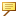About Bend Allowance and Developed Length
Bend allowance calculations are used to determine the developed length of flat material required to make a bend with a specific radius and angle. These calculations are important to create an accurate flattened model. Bend allowance calculations take into account the thickness, bend radii, and bend angles of flat material. Bend allowance compensates for stretching in the area of a bend, where the material on the outside of the neutral bend axis stretches while the material on the inside of the neutral bend axis compresses. You can set default values for bend allowance for the part or override these values using a feature-specific bend allowance calculation method during board creation. Depending on the type of developable geometry, you can use one of the following methods to calculate the developed length of a board:
System-defined equation that uses either Y factor or K factor to calculate the developed length for all developable geometry
Bend table that uses a standard or customized bend table to calculate the developed length only for geometry containing arcsYou can override an incorrect value for developed length by modifying the value or by using a customized bend table.
You can use the following system-defined equation to calculate developed length:
L = (Π/2 x R + Y factor x T) Θ/90
Where: L =
Developed length
Π =
3.142
R =